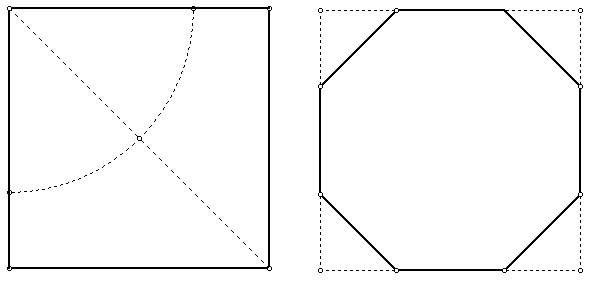#### You may also like### Diophantine N-tuples

Can you explain why a sequence of operations always gives you perfect squares?### DOTS Division

Take any pair of two digit numbers x=ab and y=cd where, without loss of generality, ab > cd . Form two 4 digit numbers r=abcd and s=cdab and calculate: {r^2 - s^2} /{x^2 - y^2}.### Sixational

The nth term of a sequence is given by the formula n^3 + 11n . Find the first four terms of the sequence given by this formula and the first term of the sequence which is bigger than one million. Prove that all terms of the sequence are divisible by 6.

# The Medieval Octagon

##### Age 14 to 16Challenge LevelMedieval stonemasons used this method to construct exact octagons in a given square window.

Open your compasses to a radius of half the diagonal of the square and construct an arc with centre one vertex of the square - mark the 2 points where the arc crosses the sides.

Do that for all 4 vertices of the square giving 8 points which are the vertices of an octagon. Is the octagon an exact regular octagon? You may wish to explore using the GeoGebra applet below.

Move the slider to rotate the yellow square. Does this help you to prove that the octagon is regular?